##1. Perspectivity

A perspectivity is a projectivity (see Projectivity.html ) fixing the points of a line (a), called the axis of perspectivity, and leaving invariant all the lines through a point A, called the center of perspectivity.
If point A is not contained in line (a), then the perspectivity is more specifically (Sophus Lie 1842-1899) called a homology.
If point A is contained in line (a), then the perspectivity is called an elation.

##2. Perspectivity through two triangles

This projective transformation is defined by fixing two perspective triangles A1A2A3 and B1B2B3, i.e. two triangles whose lines AiBi pass through a fixed point O for i=1,2,3.
The perspectivity F with this data, is uniquely defined by the requirement to fix O (F(O)=O) and map F(Ai)=Bi, for i=1,2,3. The following properties are easily proven.
 By Desargues theorem, the corresponding sides of the triangles intersect at three collinear points C1, C2, C3.
 Line (a) containing the three points {Ci} coincides with the axis of perspectivity. Point O coincides with the center of perspectivity.
 The three points {Ci} are fixed points of F. This implies that all the points of the axis are fixed by F.
 For two points X, X' and their images Y=F(X), Y'=F(X'), lines XX' and YY' intersect on the axis of F.
 A perspectivity is completely determined by giving its center O, its axis (a) and (X,Y), later being a couple of homologous points (Y=F(X)).
 Algebraically, perspectivities are characterized by the fact that they have a line (a) consisting entirely of fixed points. They are represented by matrices having two real and equal eigenvalues.
In the case of homologies, property  implies that the cross ratio (O,HX,X,Y) = k is constant for all points X, Y = F(X) and HX denoting the intersection of line OX with the axis (a). k is called the coefficient of the homology.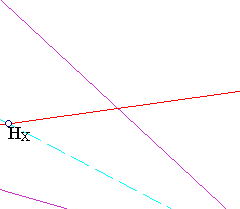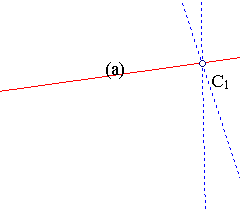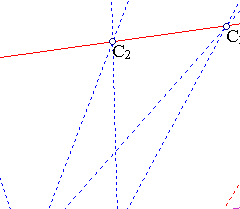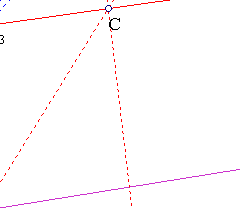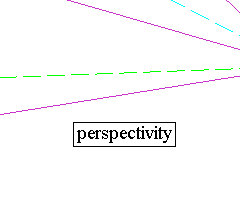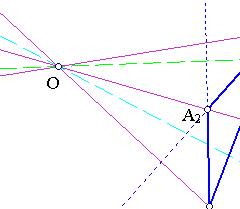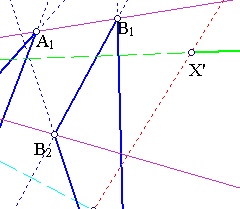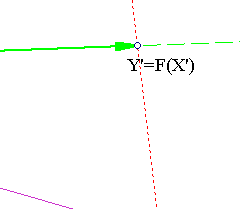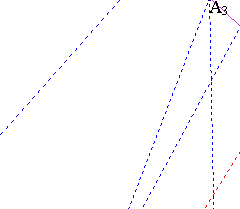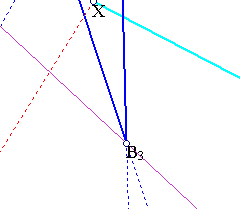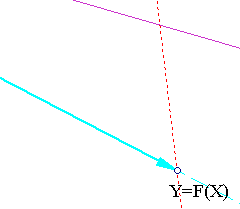Homologies with homology coefficient = -1 are called harmonic homologies or harmonic harmonic perspectivities (see Harmonic_Perspectivity.html ). Considering the euclidean plane and its projectification, a general homology, with coefficient k, maps the line parallel to (a) at distance-ratio k from it and O, to the line at infinity.
The discussion continues in the file Elation.html .

##3. The affine case

Perspectivities F of the affine plane leave in addition invariant one line: the line at infinity.
 If they are homologies, then either the center, or the axis are at infinity. In fact, if neither is at infinity, then it is easily shown that F fixes all lines through the center, hence is the identity.
 If D is not at infinity, then the axis is the line at infinity and the perspectivity is a homothety with center D.
 If D is at infinity, then the axis is left pointwise fixed and every line in the direction determined by D is left invariant. For a point X and its image Y=F(X) it is HX/HY=k a constant, where H is the intersection point of the line XD with the axis. In particular, k=-1 gives an affine reflexion.

 If they are elations, then the center O must be at infinity. Otherwise, all the lines through it being invariant, their points at infinity are fixed, hence they are on the axis and this is a contradiction.
 If the axis of the elation is the line at infinity, then every line maps to a parallel line and the lines in the direction of the center O remain invariant, hence it is a translation.
 If the axis of the elation is not the line at infinity, then it preserves again all lines in the direction of O, through which also passes the axis a. Thus all parallels to a are preserved, hence the map is a shear.